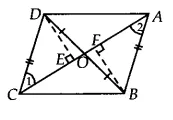Q

# In Fig. 9.25, diagonals AC and BD of quadrilateral ABCD intersect at O such that OB = OD. If AB = CD, then show that: (i) ar (DOC) = ar (AOB)

Q: 6     In Fig. , diagonals AC and BD of quadrilateral ABCD intersect at O such that. If , then show that:

(i)

[Hint: From D and B, draw perpendiculars to AC.]Views

We have ABCD is quadrilateral whose diagonals AC and BD intersect at O. And OB = OD, AB = CD
Draw DE  AC and FB ACIn DEO and  BFO
DOE = BOF [vertically opposite angle]
OED = BFO [each ]
OB = OD [given]

Therefore, by AAS congruency
DEO    BFO
DE = FB [by CPCT]

and ar( DEO) =  ar(BFO) ............(i)

Now, In  DEC and  ABF
DEC = BFA [ each ]
DE = FB
DC = BA [given]
So, by RHS congruency
DEC    BFA
1 = 2 [by CPCT]
and, ar( DEC) =  ar(BFA).....(ii)

By adding equation(i) and (ii), we get

Hence proved.

Exams
Articles
Questions